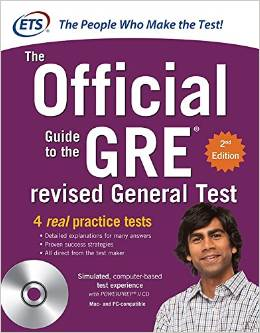GRE Keys Program coaches leverage the Facilitative Approach in order to assess the strengths and areas for improvement of each student. Students arrive at each new session with a list of the homework questions that they either answered incorrectly, or that took them ‘too long’ to answer. While students work through questions on the board, their MathSP coach facilitates a discussion that enables students to find the best solutions and understand their mistakes. Through the expert guidance of the MathSP coach and the input of their peers, students work through missed questions to not only correct their mistakes, but to also gain a solid understanding of the concepts at the center of each GRE question.

### The Key Program Course Information

• Intense foundation review of important Math concepts (listed below)
• Real GRE problems tackled using the SP Method
• Organization of problems by concept to promote Concept-based Learning
• Extensive homework assignments each session
• Homework review using the Facilitative Approach
• Online practice tests to encourage familiarity with the computer-based format and scoring model
• Timed practice sessions

### The Key Program Course Outline

The Keys Program consists of eight 3-hr sessions emphasizing the Quantitative problems from “The Official Guide to the GRE Revised General Test,” by the official test makers, ETS. Using this text and supplementary texts, students work through real GRE problems that are similar to those they are likely to see on their test.

Sessions are organized as follows:

• Session 1: Arithmetic Part I
• Session 2: Arithmetic Part II
• Session 3: Algebra Part I
• Session 4: Algebra Part II
• Session 5: Statistics
• Session 6: Geometry Part I
• Session 7: Geometry Part II
• Session 8: Data Analysis and Interpretation Sets

### The Keys Program Concept

The Quantitative section of the GRE tests your knowledge of a finite number of Math concepts. Mastering these concepts is critical to scoring your highest on the test. In the Keys Program, our coaches teach the Math concepts listed below that are required for your success on the GRE.

The concepts can be categorized into four groups:

• arithmetic
• algebra & statistics
• geometry
• data analysis and interpretation

Concepts include:

##### Arithmetic

Number Theory, Properties of Integers, Fractions, Decimals, Divisibility Rules, Prime Numbers, Even and Odd Numbers, Positive and Negative Numbers, Consecutive Integers, Number Lines, Digits, Factors and Multiples, Factor Trees, Order of Operations, Percentages, Ratios, Proportions, Squares and other Powers, Square Roots

##### Algebra & Statistics

Exponents, Solving Equations, Substitution, FOIL, Factoring, Inequalities, Applied Algebra, Translating Math Sentences into Equations, Mean, Median, Sets, Graphs of Equations, Slope, Intercepts

##### Geometry

Lines and Angles, Circles, Area, Circumference, Triangles, Isosceles, Equilateral, Special Right Triangles, Pythagorean Theorem, Perimeter, Quadrilaterals, Integrated Shapes, Volume, Coordinate Geometry

##### Data Representation and Interpretation

Probability, Mean, Median, Mode, Range, Standard Deviation, Percentiles, Interpretation of Data in Graphs and Tables

### The Keys Program Course Materials

##### Course Materials Include:• “The Official Guide to the GRE revised General Test,” by the ETS
• Math foundation review books (optional)